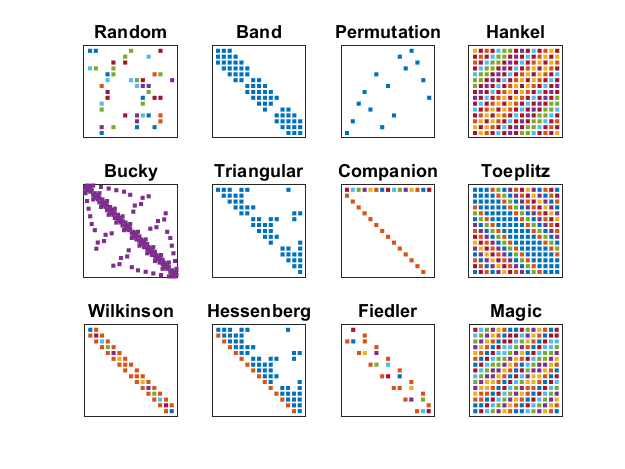# MiniGallery, Sampler of MATLAB Test Matrices

MATLAB has dozens of test matrices. Here are a few.

### Contents#### Mini_Gallery

• Random. A = sprand(n,n,0.25). Random sparse, density = 0.25.
• Bucky. A = bucky. Sparse connectivity graph of the geodesic dome, the soccer ball, and the carbon-60 molecule.
• Wilkinson. A = wilkinson(n). Wn+. Nearly equal double eigenvalues.
• Band. A = triu(tril(A,2),-2). Elements near diagonal.
• Triangular. A = triu(A). Elements on and above diagonal.
• Hessenberg. A = triu(A,-1). Upper triangular plus one subdiagonal. See schur.
• Permutation. A = sparse(randperm(n),1:n,1). One +1 in each row and column.
• Companion. c = charpoly(A); A = [-c(2:end); eye(n-1,n)]. Traditional companion matrix.
• Fiedler. c = charpoly(A); A = fiedler(-c(2:end)). Fiedler companion matrix.
• Hankel. A = flip(gallery('toeppd',n)). Constant antidiagonals.
• Toeplitz. A = gallery('toeppd',n). Constant diagonals.
• Magic. A = magic(n). Magic square.

#### Software

Published with MATLAB® R2023a

|